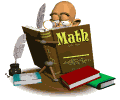•

These websites have games and activities to help you learn and practice your skills needed to become awesome students!!! Have fun, and keep yourself challenged.

 MathLink - description Rating Math FlashPractice math facts and see if you can beat the clock with addition, subtraction, multiplication, division and mixed practice.Math MagicianPractice math facts (addition, subtraction, multiplication and division and mixed operations) with timer.Elapsed time practiceTen questions to answer and self check on elapsed time.Elapsed TimeUse clocks to figure out elapsed time and check your answers.Elapsed TimeMatching, flash cards and concentration games to help with elapsed time. Elapsed TimePractice with a digital or analog clock how to figure out elapsed time.Prime and Composite GamePractice identifying prime and composite numbers.A+ MathPractice math facts with interactive flashcards and math games. Worksheets can be printed to practice math offline. A homework helper section to check your homework computation.AAA MathExcellent math resource for home. Choose the grade level, and an entire year's worth of math concepts is at your fingertips. This site explains concepts and provides multiple practice opportunities.Baseball MathBaseball lovers should try this one! Practice math skills of addition, subtraction, multiplication, division and mixed practice. Levels range from easy to superbrain!!Cool MathReally cool math site!! Lessons provided on various math skills, with practice and game opportunities. What is really cool is the math dictionary which has definitions and pictures to explain many math concepts from arithmetic to algebra.Fraction Flag GameCreate a flag with different fractional colored parts.Fraction MatchGreat easy matching game with fractions!! Matching pictures to fractions. Make sure you scroll down to read the directions.Fraction Match with wordsMatching fraction pictures with words.Math DictionaryA dictionary for kids in grades K-6 on unfamiliar math terms! Excellent source to get any math definition.Math Fact CafePrintable math worksheets for many math skills (i.e., whole number computation, fractions with reducing, decimals, percents)Math Fact ChallengeOne minute timed tests for addition, subtraction, multiplication, and division.MultiplicationGreat site for practicing multiplication facts.Multiplication.comPractice your math facts with fun games!Perimeter and AreaPractice calculating perimeter and area of different shapes